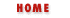#Chapter 4Expressions and Operators## 4.5 String Operators

As we've noted in the previous sections, there are several operators that have special effects when their operands are strings.

The + operator concatenates two string operands. That is, it creates a new string that consists of the first string followed by the second. Thus, for example, the following expression evaluates to the string "hello there":

```"hello" + " " + "there"
```

And the following lines produce the string "22":

```a = "2"; b = "2";
c = a + b;
```

The <, <=, >, and >= operators compare two strings to determine what order they fall in. The comparison uses alphabetical order. Note, however, that this "alphabetical order" is based on the ASCII or Latin-1 (ISO8859-1) character encoding used by JavaScript. In this encoding, all capital letters come before (are "less than") all lowercase letters, which can cause unexpected results. It means, for example, that the following expression evaluates to true:

```"Zoo" < "aardvark"
```

The == and != operators work on strings, but, as we've seen, these operators work for all data types, and they do not have any special behavior when used with strings.

The + operator is a special one--it gives priority to string operands over numeric operands. As noted earlier, if either operand to + is a string (or an object) the the other operand (or both operands) will be converted to strings and concatenated, rather than added. On the other hand, the comparison operators only perform string comparison if both operands are strings. If only one operand is a string, JavaScript attempts to convert it to a number. The following lines illustrate:

```1 + 2         // Addition. Result is 3.
"1" + "2"     // Concatenation. Result is "12".
"1" + 2       // Concatenation; 2 is converted to "2". Result is 12.
11 < 3        // Numeric comparison. Result is false.
"11" < "3"    // String comparison. Result is true.
"11" < 3      // Numeric comparison; "11" converted to 11. Result is false.
"eleven" < 3  // Causes error because "eleven" can't be converted to a number.
```

Finally, it is important to note that when the + operator is used with strings and numbers, it may not be associative. That is, the result may depend on the order in which operations are performed. This can be seen with examples like this:

```s = 1 + 2 + "blind mice";        // yields "3 blind mice"
t = "# of blind mice: " + 1 + 2; // yields "# of blind mice: 12"
```
The reason for this surprising difference in behavior is that the + operator works from left to right, unless parentheses change this order. Thus the two lines above are equivalent to these:

```s = (1 + 2) + "blind mice";          // 1st + yields number; 2nd yields string
t = ("# of blind mice: " + 1) + 2;   // both operations yield strings
```Comparison OperatorsLogical Operators